Project 2 : Pb-Lite Boundary Detection

Paul Sastrasinh (psastras)

Summary

The goal of this project was to create a boundary detector similar to one outlinied in Arbelaez et al. 2011. This boundary detector improves over Canny and Sobel edge detection by reasoning about texture data, and not just brightness gradients. The first step of the boundary detection is to run either a Canny or Sobel edge detector as the baseline edge detection. The problem with these detectors is that it detects boundaries in places where there should not necessary be boundaries, particulary in regions of similar texture, such as a field of grass. To filter out these false boundaries, a texton map is created and used to measure the similarity of a one pixel's texture to another. Once the texton map has been created, the gradient of the texton map is measured in different directions at each pixel is measured to determine if a boundary should be kept or not. The larger the gradient, the higher the probability of boundary. The output is a boundary probability map, which is then combined with the Sobel or Canny baseline boundary to produce the final result.

Texton Map Creation

To create the texton map, a filter bank consisting of DoG filters at different scales and orientations is created (see the image below). In my implementation the DoG filters are created by convolving a Sobel filter with a Gaussian.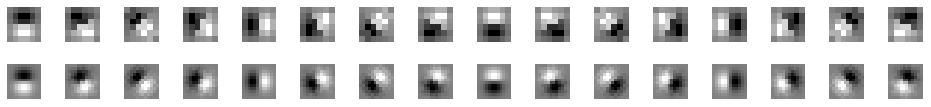Texton Filter Bank

Once the filter bank has been created, the image is filtered by each of the filters in the filter bank. The results are then clustered using k-means, assigning each pixel into one of k different textures to create a texton map of the image.

The gradient of the brigtness and texture is created by convolving a binned brightness image and the texton map with oriented half discs are used in two different directions. The disc masks are in the image below. The gradient is determined as the chi-squared difference between the histograms computed from the binary half discs.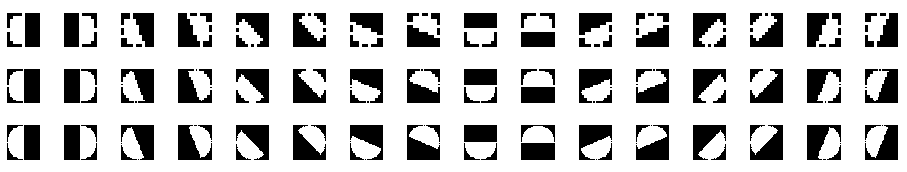Half Disc Masks

Extensions

Cg

Besides texture gradient (tg) and brightness gradient (bg), I also incorporated color gradient (cg). Each image is first converted into Lab color space. Then, for the brighness gradient, the L channel is used (instead of the grayscale image). The color gradient is then created in a similar method using the a and b channels. This provided a minor improvement in performance over Canny and Sobel baselines.

Filter Banks

Instead of using a filter bank of oriented Gaussian derivatives, the filter bank was changed to the LM filter bank as described in Representing and Recognizing the Visual Appearance of Materials using Three-dimensional Textures (Leung, Malik 2001). The filter bank consists of 48 different filters, including 36 oriented elongated Gaussian first and second derivative filters at three scales and 6 orientations (such that sigma_x = 3 sigma and sigma_y = sigma), 8 LoG filters at different scales, and 4 Gaussians at different scales. Sigma was chosen to be sigma = [sqrt(2) 2 2*sqrt(2) 4]. The filter bank is below.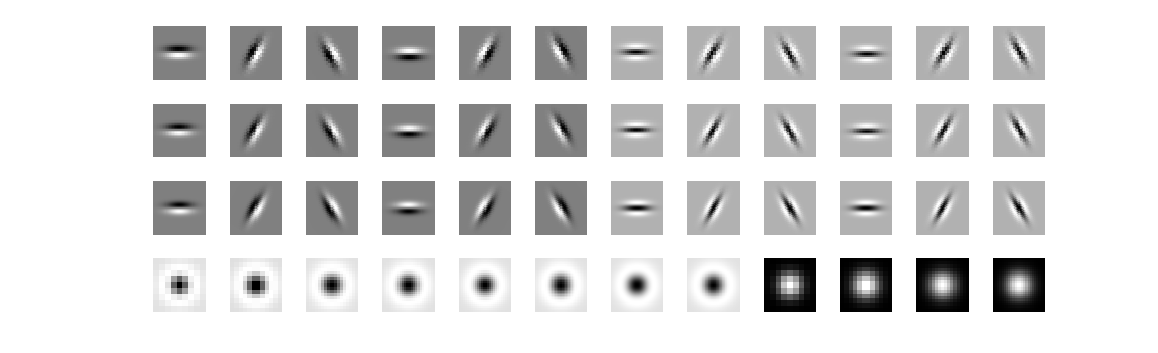LM Texton Filter Bank

I also experimented with rotation invariant fiter banks. By taking the maximal response of each set of orientations, the filter banks can be made invariant to orientation. Furthermore, this reduces the number of dimensions of the texton data by number of scales x number of orientations. This didn't really improve anything, in fact, it seemed to get a little worse.

In the final implementation I changed the LM filter bank by removing the circular Gaussian filters, and keeping sigma x and sigma y equivalent. The finalized filter bank is below.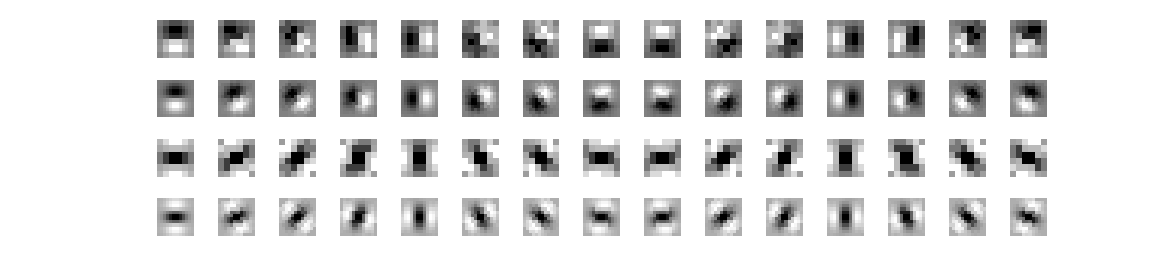Final Filter Bank Consisting of Symmetric DoG and 2nd order DoG Filters

Clustering

I found the MATLAB's kmeans implementation, while slower yielded better results (maybe it's somewhat more robust than the provided kmeans implementation?).

pB Feature Combining

I combine the gradients according to equation (10) in Arbelaez et al. 2011, which yields slightly better results after the alpha weights have been modified to place more emphasis on the texture gradient.

A Better Baseline

Instead of using a Canny or Sobel baseline, I added another baseline, based on the color compass edge detector. After looking at the results, I felt that an over complete edge detector would do better than a less sensitive detector, since the texton and other gradient maps should be able to filter out the actual non edges. However, simply turning up the threshold on the Canny detector resulted in far too many non edges (there were just as many or more new non edges as there were weaker edges). The color compass edge detector (Ruzon and Tomasi, 1999) improves upon the Canny edge detector by trying to utilize color gradients and removing vector quantization / binning. The end result is an edge detector that can detect edges which Canny cannot and localize them more accurately in scale space.

Interestingly, running the color compass edge detector versus the Canny edge detector showed no significant difference in OIS/ODS statistics for the 200 image dataset. However, combining the new color copmass edge detector with the texton and Lab gradient maps provided a significant boost in boundary detection (see the F statistics below for the new baseline and the resulting pB).

../data/baseline3tmp/
Boundary
ODS: F( 0.68, 0.51 ) = 0.58   [th = 0.44]
OIS: F( 0.74, 0.54 ) = 0.63
Area_PR = 0.53
../data/mypbtmp/
Boundary
ODS: F( 0.66, 0.66 ) = 0.66   [th = 0.27]
OIS: F( 0.72, 0.64 ) = 0.67
Area_PR = 0.64

Results

The probability of boundary is determined using the gradients according to eqn () and then dot multiplying the result with the appropriate baseline.

Detection results are presented below for different combinations of paramaters. Note that baseline1 corresponds to Sobel, baseline2 corresponds to Canny, baseline3 corresponds to compass, and mypbtmp corresponds to the final pB result.

Canny Baseline with Regular Filter Bank (k=32,64)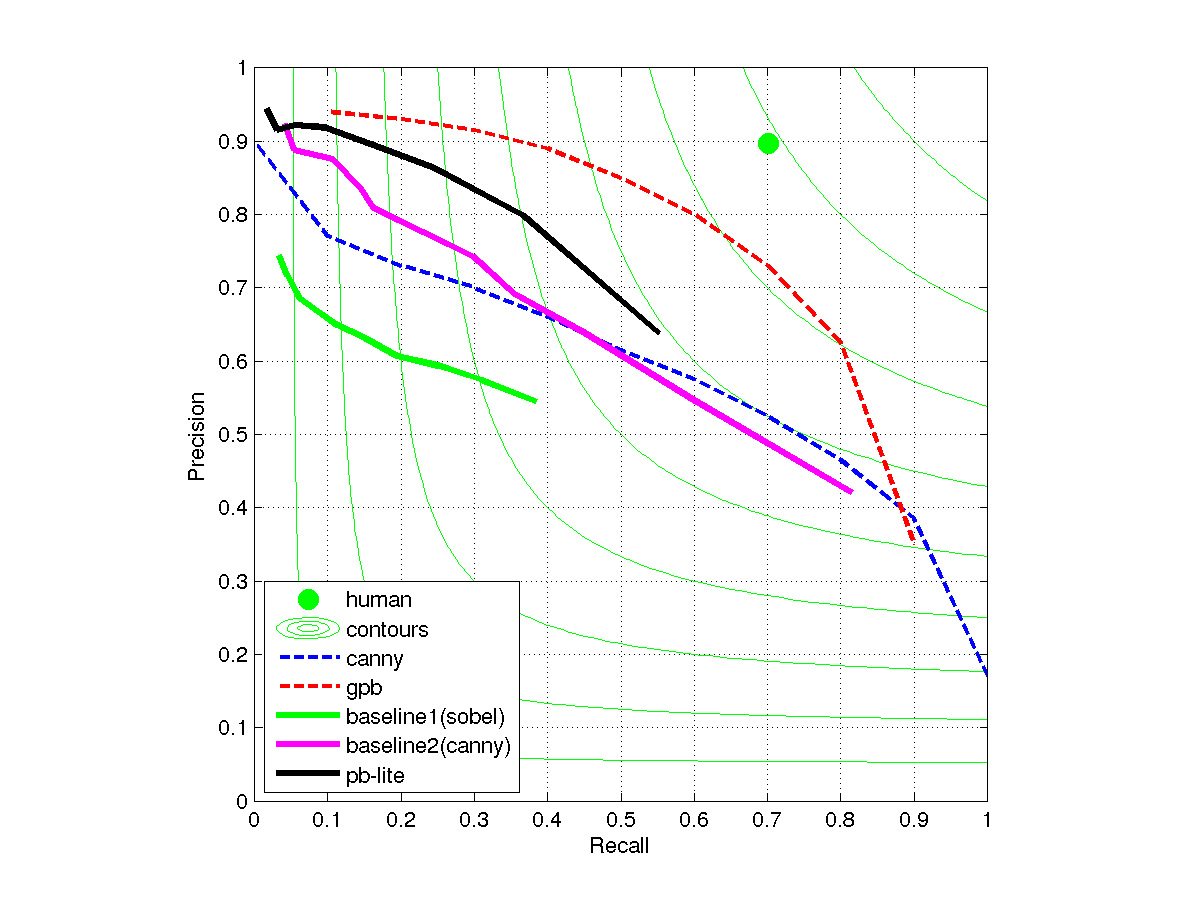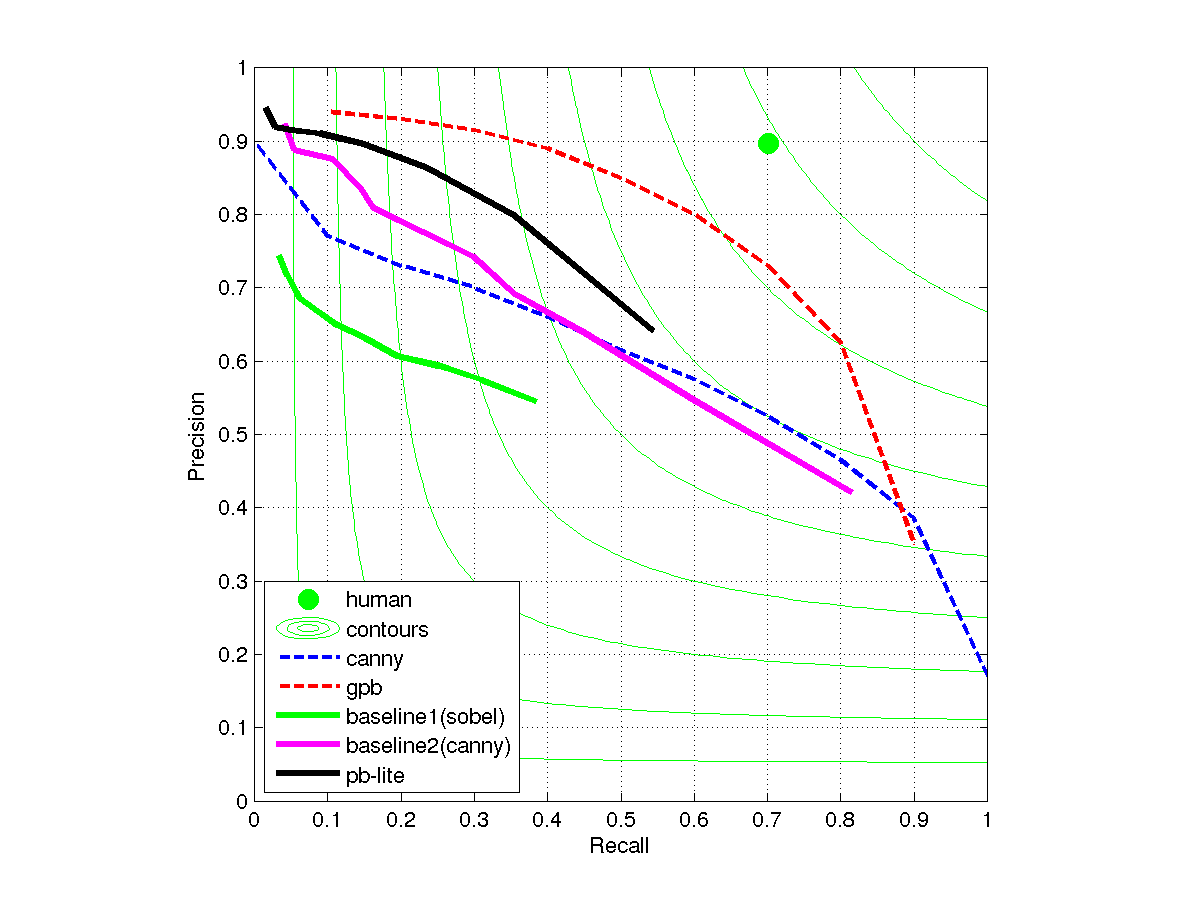Sobel Baseline with Regular Filter Bank (k=32,64)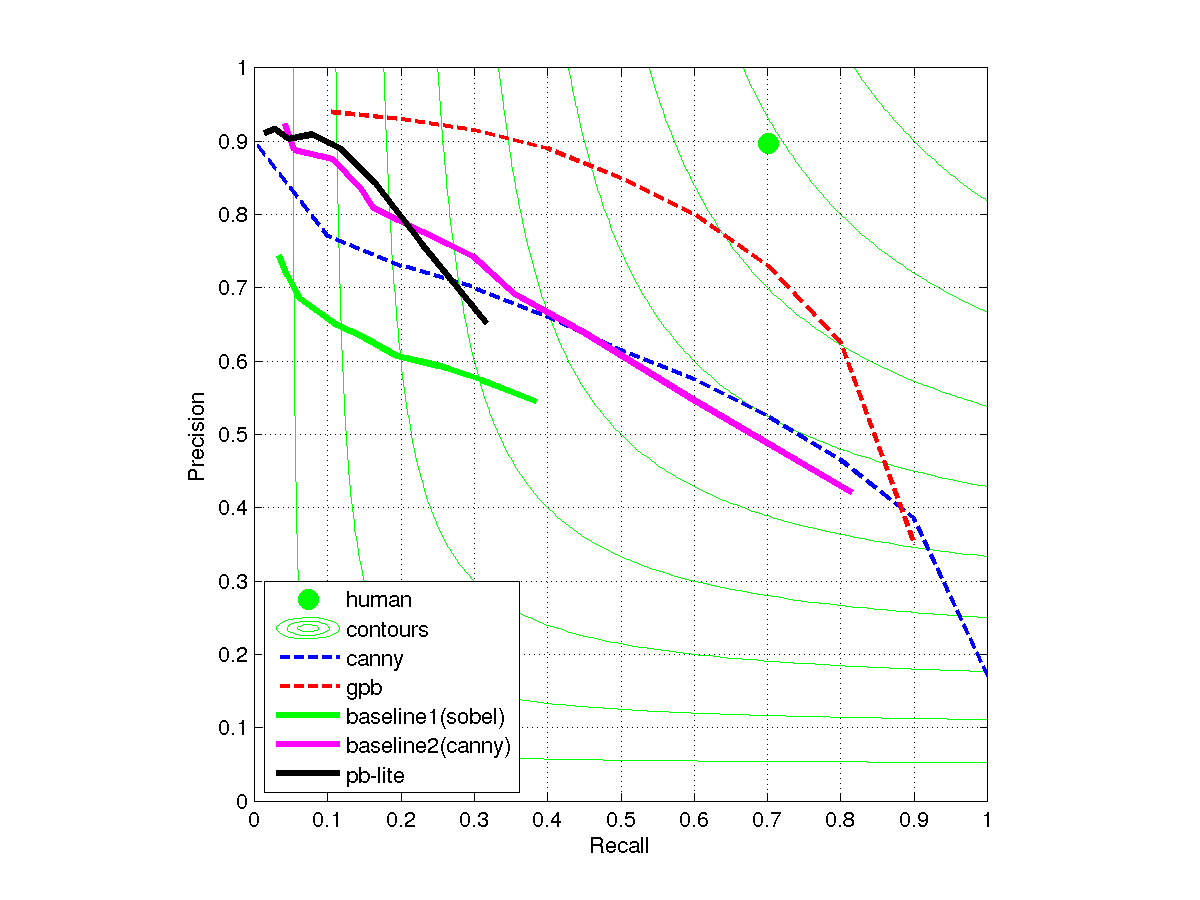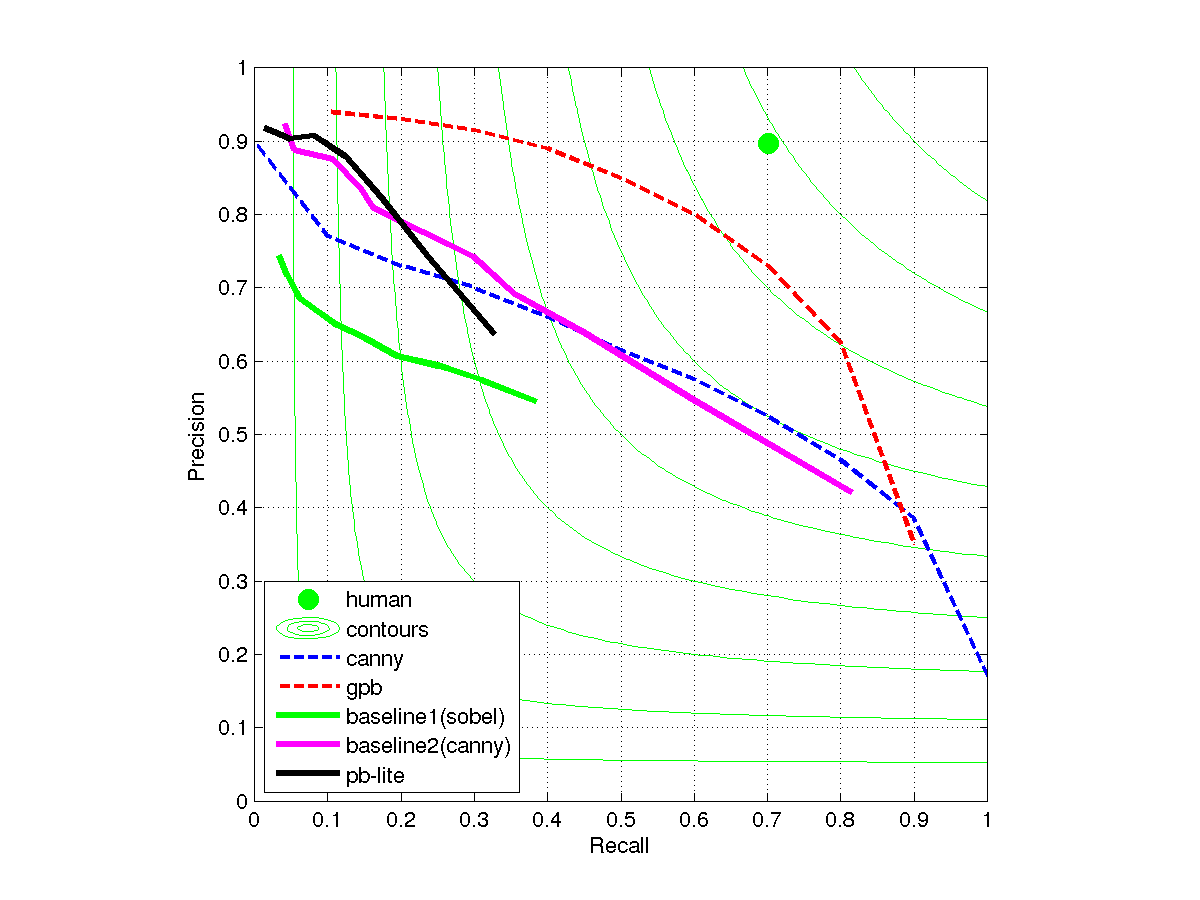Canny Baseline with Extended Filter Bank (k=32,n=10)

../data/baseline2tmp/
Boundary
ODS: F( 0.70, 0.54 ) = 0.61   [th = 0.18]
OIS: F( 0.74, 0.53 ) = 0.62
Area_PR = 0.48
../data/mypbtmp/
Boundary
ODS: F( 0.67, 0.60 ) = 0.63   [th = 0.10]
OIS: F( 0.66, 0.61 ) = 0.63
Area_PR = 0.55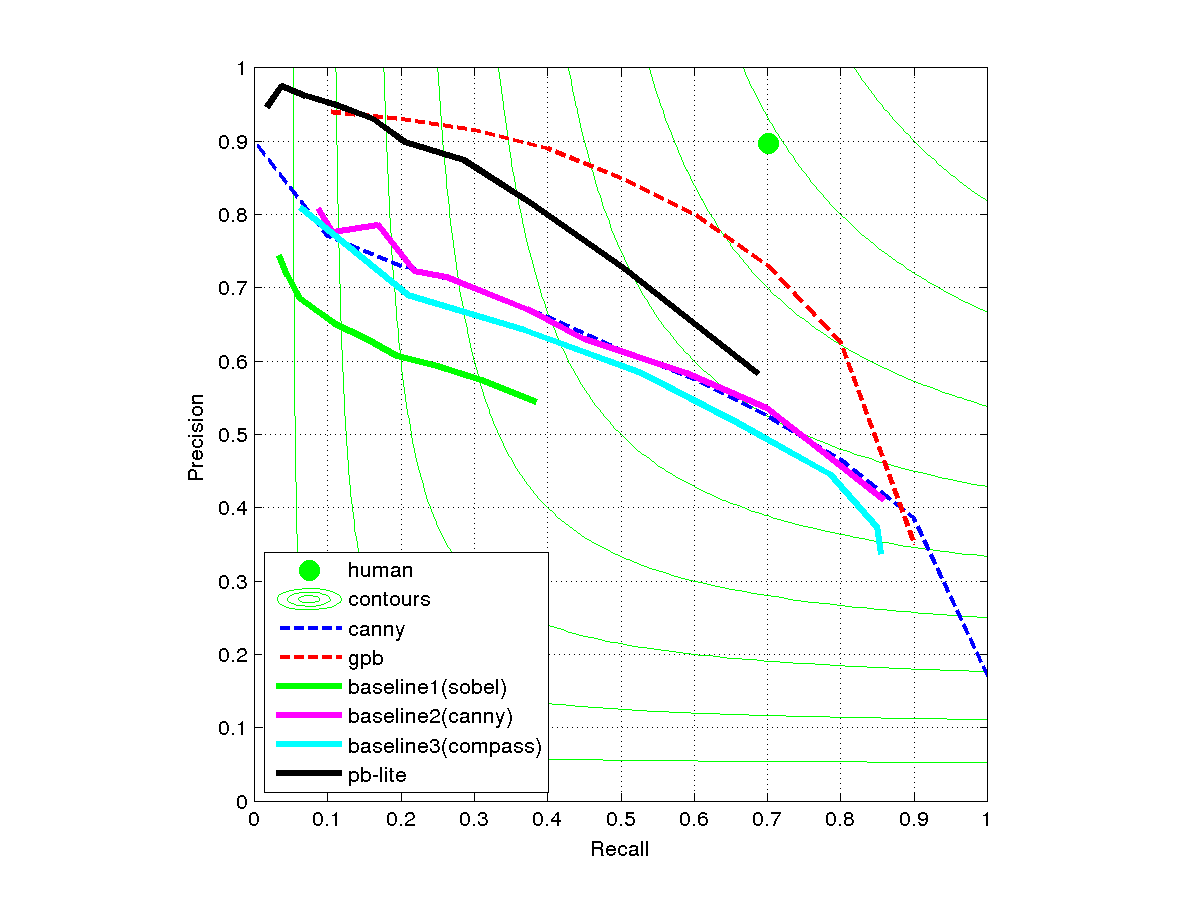Color Compass Baseline with Extended Filter Bank(k=32,n=10,200)

n=10
../data/baseline3tmp/
Boundary
ODS: F( 0.68, 0.51 ) = 0.58   [th = 0.44]
OIS: F( 0.74, 0.54 ) = 0.63
Area_PR = 0.53

../data/mypbtmp/
Boundary
ODS: F( 0.66, 0.66 ) = 0.66   [th = 0.27]
OIS: F( 0.72, 0.64 ) = 0.67
Area_PR = 0.64
n=200
../data/baseline3tmp/
Boundary
ODS: F( 0.71, 0.58 ) = 0.64   [th = 0.50]
OIS: F( 0.76, 0.59 ) = 0.66
Area_PR = 0.59

../data/mypbtmp/
Boundary
ODS: F( 0.70, 0.61 ) = 0.65   [th = 0.21]
OIS: F( 0.73, 0.63 ) = 0.67
Area_PR = 0.64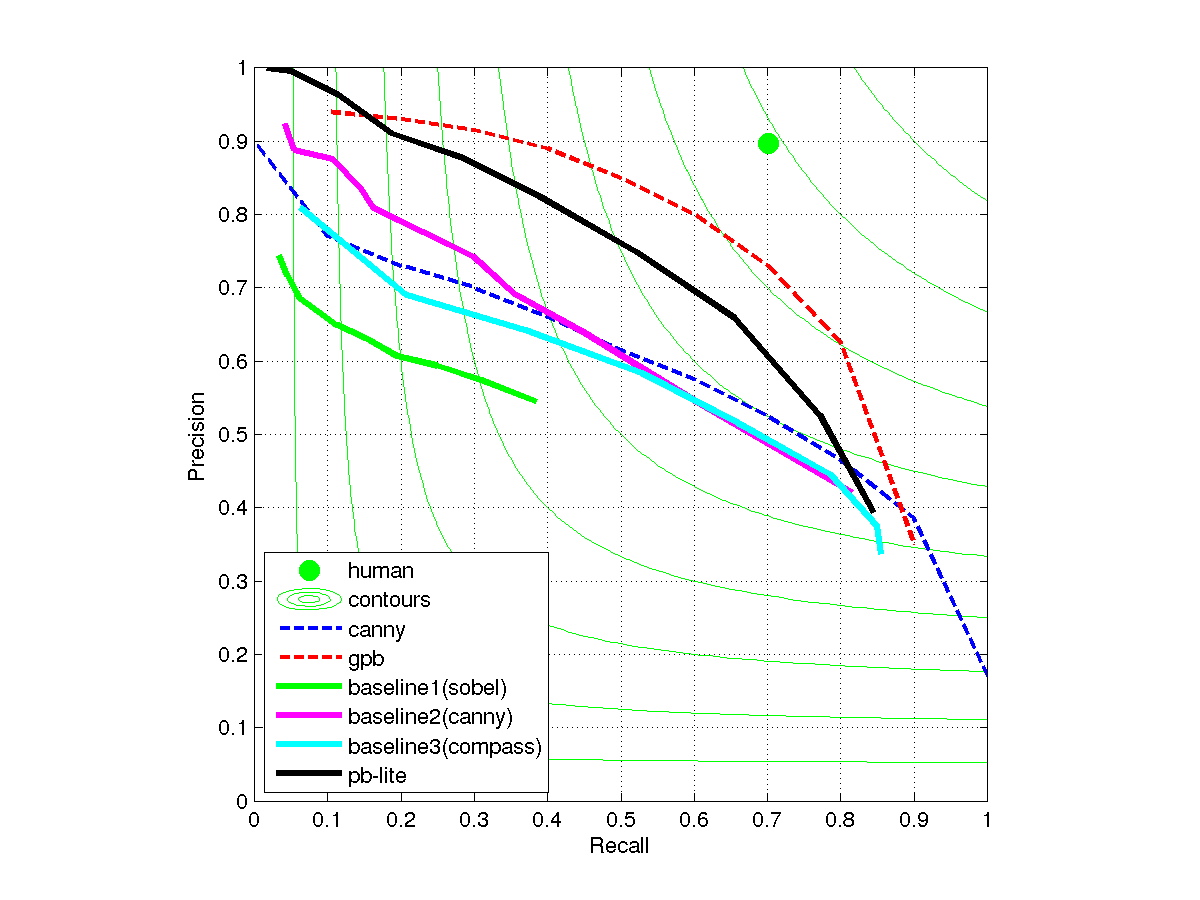Paul Sastrasinh 2011